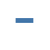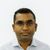# New to Qlik Sense

If you’re new to Qlik Sense, start with this Discussion Board and get up-to-speed quickly.

Announcements
cancel
Showing results for
Did you mean:Contributor III

## Set Expression comparing date fields with Count is not working

I have this expression based on a calculated field (expdate) but it isn't working. I want to count records that have expired. Instead this is just showing me all records in the dataset. I expected that expiration dates that have not occurred yet (there are several) would be excluded from this count. Is the syntax here correct at least (I suspect I need to do something w/ the date formats perhaps)?

=Count({<[expdate]={"<=\$(Today())"}>}[service_id])

Labels (1)
• ### Qlik Sense

1 Solution

Accepted SolutionsMVP

Try one of these,

``````=Count({<[expdate]={"<=\$(=Today())"}>}[service_id])
=Count({<[expdate]={"=Date([expdate])<=\$(=Today())"}>}[service_id])
=Count({<[expdate]={"=Date([expdate])<=Today()"}>}[service_id])``````
2 RepliesMVP

Try one of these,

``````=Count({<[expdate]={"<=\$(=Today())"}>}[service_id])
=Count({<[expdate]={"=Date([expdate])<=\$(=Today())"}>}[service_id])
=Count({<[expdate]={"=Date([expdate])<=Today()"}>}[service_id])``````Contributor III
Author

I actually used the top one but ended up taking the "=" out (I was just confused about what I wanted). Thanks for your assistance!

``=Count({<[expdate]={"<\$(=Today())"}>}[service_id])``

That did the trick